# SORTBY Function Examples – Excel

This tutorial demonstrates how to use the SORTBY Function in Excel to sort a list of values based on a list of corresponding  values.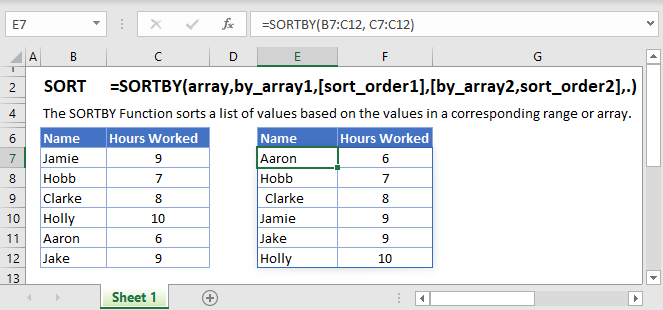## SORTBY Function Overview

The SORTBY Function is an extension of the SORT Function. It makes it easier to order values in an array based on the values in a corresponding array or range.

To use the SORTBY Excel Worksheet Function, select a cell and type: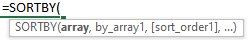(Notice how the formula inputs appear)

## SORTBY function Syntax and inputs:

`=SORTBY(array, by_array1, [sort_order1], [by_array2, sort_order2],…)`

array – The array or range to sort.

by_array1 – The array or range to sort on.

[sort_order1] (optional) – The order to use for sorting. 1 for ascending, -1 for descending. When omitted it defaults to 1 i.e.  ascending order.

[byarray2] (optional) – The second array or range to sort on.

[sort_order2] (optional) – The order to use for sorting. 1 for ascending, -1 for descending. Default is ascending.

# How to use the SORTBY  Function

## To sort values in ascending order

To sort the employees in A2:A7 in the ascending order of the hours they worked in B2:B7, we enter the following formula in D2:

`=SORTBY(A2:B7, B2:B7)`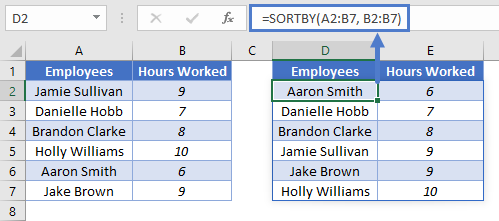If we only want to return the list of sorted employees, we enter the following formula in D2:

`=SORTBY(A2:A7, B2:B7)`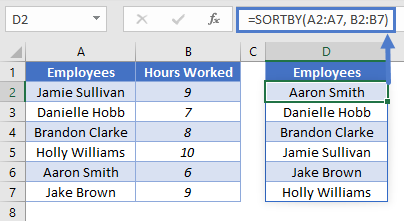You’ll notice that we’ve set the [array] argument to A2:A7 as we only want the first column returned.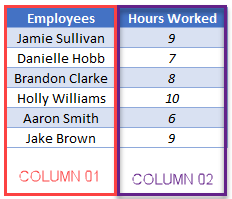## To sort values in descending order

To sort the employees in A2:A7 in the descending order of the hours they worked, we enter the following formula in D2:

`=SORTBY(A2:B7,B2:B7, -1)`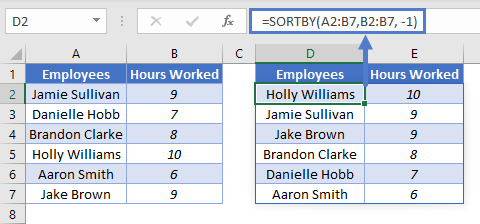You’ll notice that the third argument [sort_order] is set to -1 so as to sort the number of hours in descending order. When set to 1 the formula sorts the hours in ascending order as shown in the previous section.

## To sort values by multiple columns

To sort the employees in A2:A7 by the second column i.e hours worked and then by the first column i.e employees, we enter the following formula in D2:

`=SORTBY(A2:B7, B2:B7, 1, A2:A7, 1)`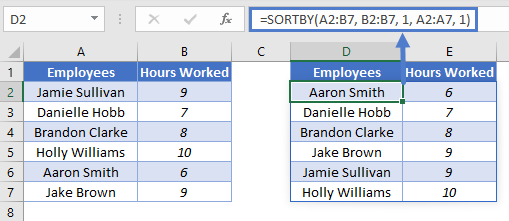## Issues

### #SPILL!

This error occurs when there is a value in the Spill Range i.e. the range where the SORTBY Function places its results.

To correct this error, clear the range that Excel highlights.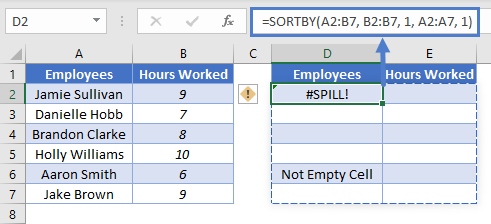### SORTBY Tips and Tricks

1. Ensure that the arguments [array]  and [byarray] have the same number of rows:

#### This will work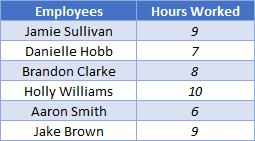#### This will NOT work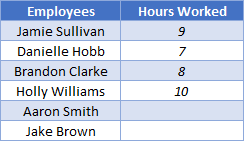2. The [sort_order] can only either be 1(ascending) or -1(descending), when no value is given for this argument the SORTBY function defaults to sorting in ascending order.

3.Ensure that the cells below the input cell are blank to avoid the Spill Error, learn more about the Spill Error ‘here’ — add link to Intro to DAFs.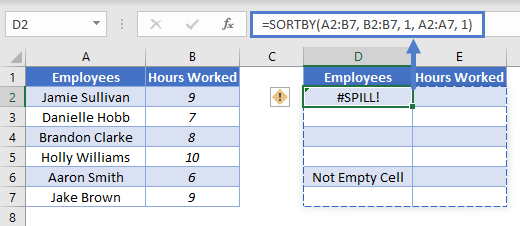4. SORTBY can be used with other Dynamic Array Functions such as FILTER to create more versatile formulas.

5. You can use the SORT Function in place of the SORTBY Function to sort values by another range or array.

`=SORT(A2:B7,2)`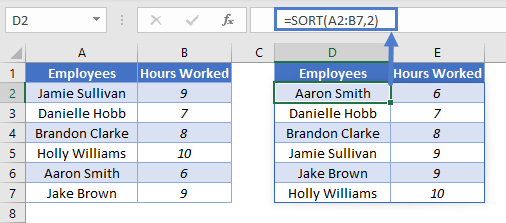The only limitation here is that we have to include the column we use to sort in the output when we use SORT.